# Dihedral angle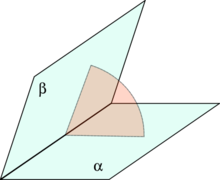Angle between two planes (α, β, green) in a third plane (pink) which cuts the line of intersection at right angles

A dihedral angle is the angle between two intersecting planes. In chemistry it is the angle between planes through two sets of three atoms, having two atoms in common. In solid geometry it is defined as the union of a line and two half-planes that have this line as a common edge. In higher dimension, a dihedral angle represents the angle between two hyperplanes.

## Definitions

A dihedral angle is an angle between two intersecting planes on a third plane perpendicular to the line of intersection.

A torsion angle is a particular example of a dihedral angle, used in stereochemistry to define the geometric relation of two parts of a molecule joined by a chemical bond.

## Dihedral angles in stereochemistry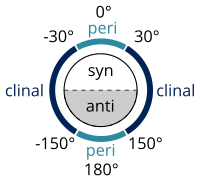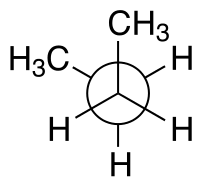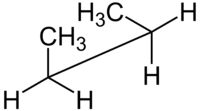Configuration names syn n-Butane Newman projection syn n-Butane sawhorse projection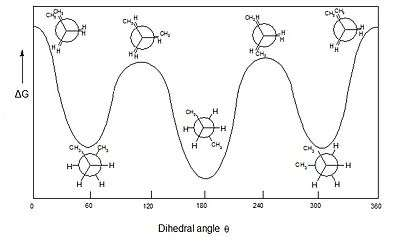Free energy diagram of butane as a function of dihedral angle.

In stereochemistry every set of three (not co-linear) atoms of a molecule defines a plane. When two such planes intersect, the angle between them is a dihedral angle. Dihedral angles are used to specify the molecular conformation. Stereochemical arrangements corresponding to angles between 0° and ±90° are called syn (s), those corresponding to angles between ±90° and 180° anti (a). Similarly, arrangements corresponding to angles between 30° and 150° or between −30° and −150° are called clinal (c) and those between 0° and ±30° or ±150° and 180° are called periplanar (p).

The two types of terms can be combined so as to define four ranges of angle; 0° to ±30° synperiplanar (sp); 30° to 90° and −30° to −90° synclinal (sc); 90° to 150° and −90° to −150° anticlinal (ac); ±150° to 180° antiperiplanar (ap). The synperiplanar conformation is also known as the syn- or cis-conformation; antiperiplanar as anti or trans; and synclinal and anticlinal as gauche or skew.

For example, with n-butane two planes can be specified in terms of the two central carbon atoms and either of the methyl carbon atoms. The syn-conformation shown above, with a dihedral angle of 60° is less stable than the anti-configuration with a dihedral angle of 180°.

For macromolecular usage the symbols T, C, G+, G, A+ and A are recommended (ap, sp, +sc, −sc, +ac and −ac respectively).

## Dihedral angles of proteinsDepiction of a protein, showing backbone dihedral angles

A Ramachandran plot (also known as a Ramachandran diagram or a [φ,ψ] plot), originally developed in 1963 by G. N. Ramachandran, C. Ramakrishnan, and V. Sasisekharan, is a way to visualize energetically allowed regions for backbone dihedral angles ψ against φ of amino acid residues in protein structure. The figure at right illustrates the definition of the φ and ψ backbone dihedral angles (called φ and φ′ by Ramachandran).

In a protein chain three dihedral angles are defined as φ (phi), ψ (psi) and ω (omega), as shown in the diagram. The planarity of the peptide bond usually restricts ω to be 180° (the typical trans case) or 0° (the rare cis case). The distance between the Cα atoms in the trans and cis isomers is approximately 3.8 and 2.9 Å, respectively. The cis isomer is mainly observed in Xaa–Pro peptide bonds (where Xaa is any amino acid).

The sidechain dihedral angles tend to cluster near 180°, 60°, and −60°, which are called the trans, gauche+, and gauche conformations. The stability of certain sidechain dihedral angles is affected by the values φ and ψ. For instance, there are direct steric interactions between the Cγ of the side chain in the gauche+ rotamer and the backbone nitrogen of the next residue when ψ is near -60°.

## Calculation of a dihedral angle

When the two intersecting planes are described in terms of Cartesian coordinates by the two equationsthe dihedral angle, φ between them is given by:An alternative method is to calculate the angle between the vectors, nA and nB, which are normal to the planes..where nA · nB is the dot product of the vectors and |nA| |nB| is the product of their lengths.

Any plane can also be described by two non-collinear vectors lying in that plane; taking their cross product yields a normal vector to the plane. Thus, a dihedral angle can be defined by three vectors, b1, b2 and b3, forming two pairs of non-collinear vectors.## Dihedral angles in polyhedra

Every polyhedron, regular and irregular, convex and concave, has a dihedral angle at every edge.

A dihedral angle (also called the face angle) is the internal angle at which two adjacent faces meet. An angle of 0° means the face normal vectors are antiparallel and the faces overlap each other (Implying part of a degenerate polyhedron). An angle of 180° means the faces are parallel (like a tiling). An angle greater than 180° exists on concave portions of a polyhedron.

Every dihedral angle in an edge-transitive polyhedron has the same value. This includes the 5 Platonic solids, the 4 Kepler–Poinsot polyhedra, the two quasiregular solids, and two quasiregular dual solids.

Given 3 faces of a polyhedron which meet at a common vertex P and have edges AP, BP and CP, the dihedral angle between the faces containing APC and BPC is:## Inverse

### Converting from dihedral angles to Cartesian coordinates in chains

It is common to represent polymers backbones, notably proteins, in internal coordinates; that is, a list of consecutive dihedral angles and bond lengths. In computational structure optimization, programs flip back and forth between representations during their iterations. This can dominate the calculation time of the potential itself and in long chain molecules introduce cumulative numerical inaccuracy. While all conversion algorithms produce mathematically identical results, they differ in speed and numerical accuracy. A review of algorithmic implementation of such inversions (comparing Quaternions, Rodriguze-gibbs, and sNerf) finds the fastest and most accurate torsion to Cartesian conversion is the Natural Extension Reference Frame (NERF) method.

## References

1. Olshevsky, George. "Dihedral angle". Glossary for Hyperspace. Archived from the original on 4 February 2007.
2. IUPAC, Compendium of Chemical Terminology, 2nd ed. (the "Gold Book") (1997). Online corrected version:  (2006) "Dihedral angle".
3. IUPAC, Compendium of Chemical Terminology, 2nd ed. (the "Gold Book") (1997). Online corrected version:  (2006) "Torsion angle".
4. Anslyn, Eric; Dennis Dougherty (2006). Modern Physical Organic Chemistry. University Science. p. 95. ISBN 978-1891389313.
5. Ramachandran, G. N.; Ramakrishnan, C.; Sasisekharan, V. (1963). "Stereochemistry of polypeptide chain configurations". Journal of Molecular Biology. 7: 95–9. doi:10.1016/S0022-2836(63)80023-6. PMID 13990617.
6. Richardson, J. S. (1981). "Anatomy and Taxonomy of Protein Structures". Advances in Protein Chemistry. Advances in Protein Chemistry. 34: 167–339. doi:10.1016/S0065-3233(08)60520-3. ISBN 9780120342341. PMID 7020376.
7. Dunbrack, RL Jr.; Karplus, M (20 March 1993). "Backbone-dependent rotamer library for proteins. Application to side-chain prediction.". Journal of molecular biology. 230 (2): 543–74. PMID 8464064.
8. Dunbrack, RL Jr; Karplus, M (May 1994). "Conformational analysis of the backbone-dependent rotamer preferences of protein sidechains.". Nature structural biology. 1 (5): 334–40. PMID 7664040.
9. Blondel, Arnaud; Karplus, Martin (7 Dec 1998). "New formulation for derivatives of torsion angles and improper torsion angles in molecular mechanics: Elimination of singularities". Journal of Computational Chemistry. 17 (9): 1132–1141. doi:10.1002/(SICI)1096-987X(19960715)17:9<1132::AID-JCC5>3.0.CO;2-T.
10. "dihedral angle calculator polyhedron". www.had2know.com. Retrieved 25 October 2015.
11. Parsons, J.; Holmes, J. B.; Rojas, J. M.; Tsai, J.; Strauss, C. E. (2005), "Practical conversion from torsion space to cartesian space for in silico protein synthesis", Journal of Computational Chemistry, 26: 1063–1068, PMID 15898109